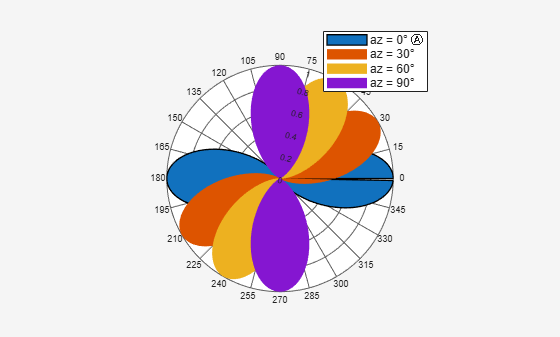# createLabels

Class: polarpattern

Create legend labels for polar plot

## Syntax

``createLabels(p,format,array)``

## Description

example

````createLabels(p,format,array)` adds the specified `format` label to each `array` of the polar plot `p`. The labels are stored as a cell array in the `LegendLabels` property of `p`.```

## Input Arguments

expand all

Polar plot, specified as a scalar handle.

Format for legend label added to the polar plot, specified as a cell array. For more information on legend label format see, `legend`.

Data Types: `char`

Values to apply to `format` , specified as an array. The values can be an array of angles or array of magnitude.

## Examples

expand all

Create a polar plot of cosine powers rotated in 30° increments. Generate a legend label for this plot.

```az = [0:359]'; a1 = abs(cosd(az).^5); a2 = abs(cosd(az - 30).^5); a3 = abs(cosd(az - 60).^5); a4 = abs(cosd(az - 90).^5); P = polarpattern([a1,a2,a3,a4],'Style','filled'); createLabels(P,'az = %d#deg',0:30:90)```